## Date and Time Formulas and Functions in Excel (part 1)

Want to display todays date or add dates, then this is for you. Want to change the date format. To change a date in a different format then go to this page (it will explain you how to write for example, 12/3/2005 or 12 March 2005 or March 12 2005, etc....The choices are infinite).

Look at part 2 for Time and hours formulas here.

Part 3 deals with weeks, weekdays and years.

Date and Time functions help you deal with dates and durations, and birthdays and days until birthday, etc….. Beware it works only from 1 January 1900….. if you want to deal with the real past, then special tricks and add in are needed.

### TODAY, DAY, MONTH, YEAR Functions

Display TODAY's date:

Just type in the cell   =TODAY()

Watch out how you can do =TODAY()+1 for exemple.....

TIP: the difference between TODAY and the function NOW(), is that NOW gives you the exact time, where TODAY only gives you the day.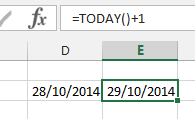A trick to make long table with a lot of thin columns with days and month and year...

Have you ever tried to get a full date into a thin column? What happens is usually that you have to use a small font and then you can not read it, or write vertically or you get the famous ####### sign.

So by using the functions DAY(cell), MONTH(cell) and YEAR(cell) you can have a nice table.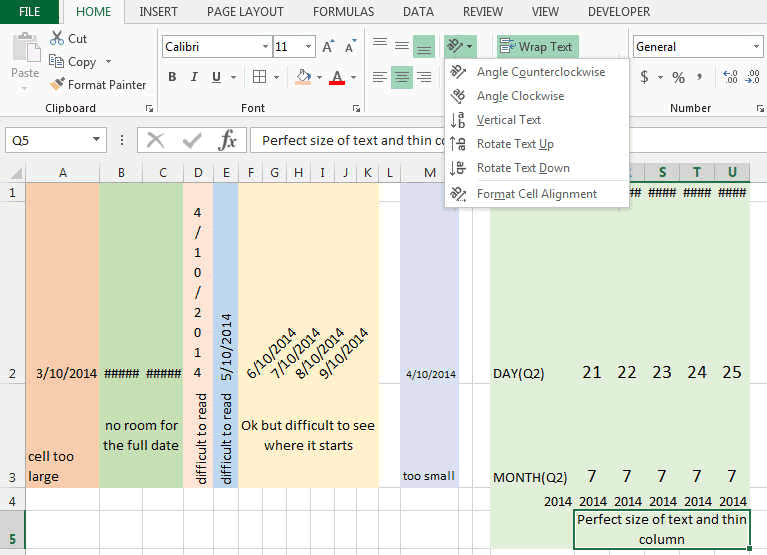Like this:

So here in B2 you will have DAY(B1)

in B3 you will have MONTH(B1)

and B4 you will have YEAR(B1)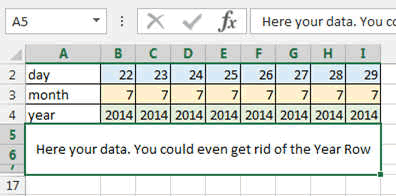Of course you can do it also by having B2, B3, B4 equal to =B1 and the changing the FORMAT of the cell like shown in our Format Page.

Both ways are fine.

### DATE, DATEVALUE, DAY360  functions

Date allows you to create a date based on 3 numbers

Use it as =DATE(Year cell, Month cell, Day cell)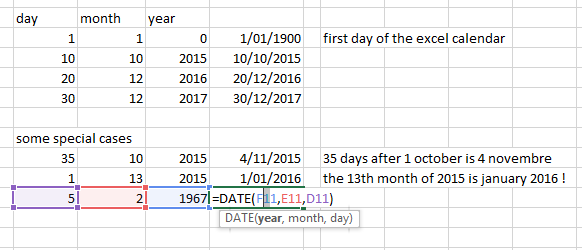DATEVALUE is creating a number (an integer) from a date in text format. This integer starts at 1 on the 1st of January 1900 and counts until

DATEVALUE("1/1/1905")     =  1948  (integer format)  or you can just set the cell in date format 1/1/1905

DATEVALUE("1/1/1900")     =  1

DATEVALUE("12/5/2015")     =  42136

Why would you use such a function? Good question. For example if you have a text field, then you can directly convert it to date.

### DAYS360(first date, second date) function

Day360 is a function that give you the difference between 2 dates based on a 360 days year.

Why would you use it? That is a specialized function used in the financial sector to simplify calculations for bonds. So use it wisely.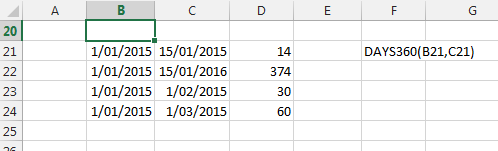Here you will find listed all the Date and Time functions so that you can have a good overview. In Bold are the ones most used and useful.

### All the Date and Time functions

DATE: The serial number of a particular date

DATEVALUE: Converts a date in the form of text to a serial number

DAY: Converts a serial number to a day of the month

DAYS360: Calculates the number of days between two dates based on a 360-day year

EDATE: The serial number of the date that is the indicated number of months before or after the start date

EOMONTH: The serial number of the last day of the month before or after a specified number of months

HOUR: Converts a serial number to an hour

MINUTE: Converts a serial number to a minute

MONTH: Converts a serial number to a month

NETWORKDAYS: The number of whole workdays between two dates

NOW: The serial number of the current date and time

SECOND: Converts a serial number to a second

TIME: The serial number of a particular time

TIMEVALUE: Converts a time in the form of text to a serial number

TODAY: The serial number of today's date

WEEKDAY: Converts a serial number to a day of the week

WEEKNUM: Converts a serial number to a number representing where the week falls numerically with a year

WORKDAY: The serial number of the date before or after a specified number of workdays

YEAR: Converts a serial number to a year

YEARFRAC: The year fraction representing the number of whole days between start_date and end_date

So that's it for the Date and Time Formulas and Functions in Excel.

Now, let's use them....